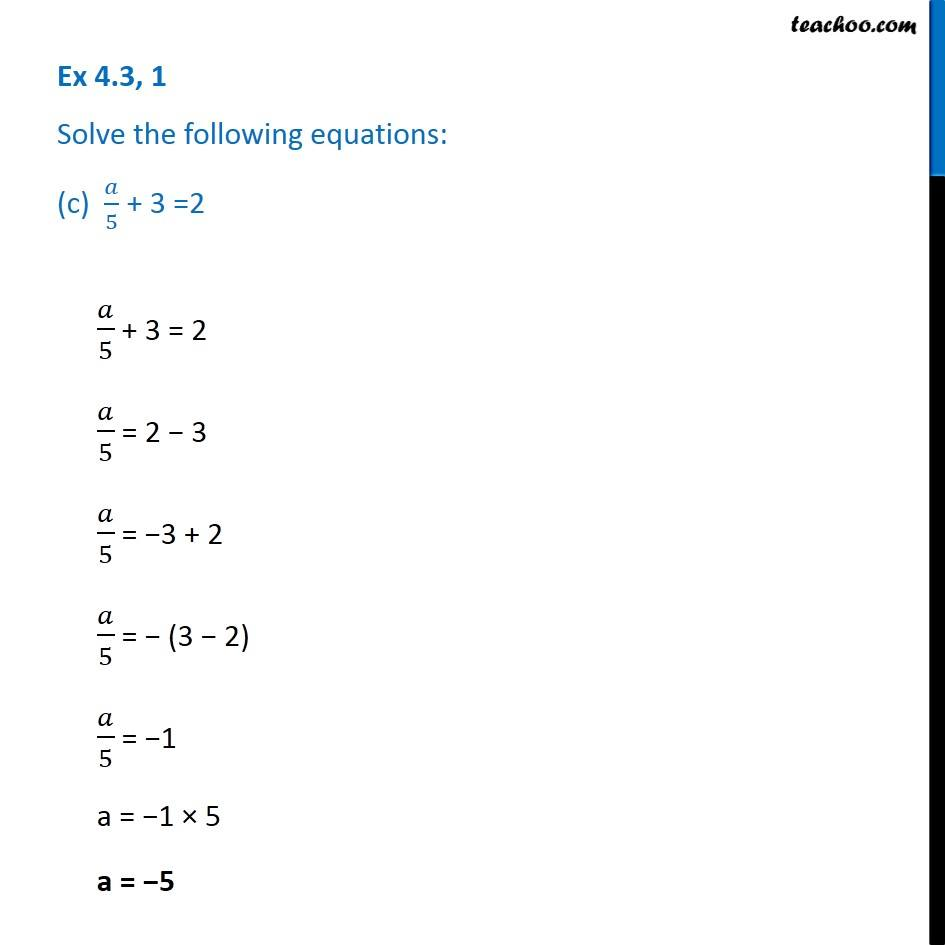Solving equations (with fractions)

Chapter 4 Class 7 Simple Equations
Serial order wiseLearn in your speed, with individual attention - Teachoo Maths 1-on-1 Class

### Transcript

Question 1 Solve the following equations: (c) 𝑎/5 + 3 =2𝑎/5 + 3 = 2 𝑎/5 = 2 − 3 𝑎/5 = −3 + 2 𝑎/5 = − (3 − 2) 𝑎/5 = −1 a = −1 × 5 a = −5